# Gravitation

## Historical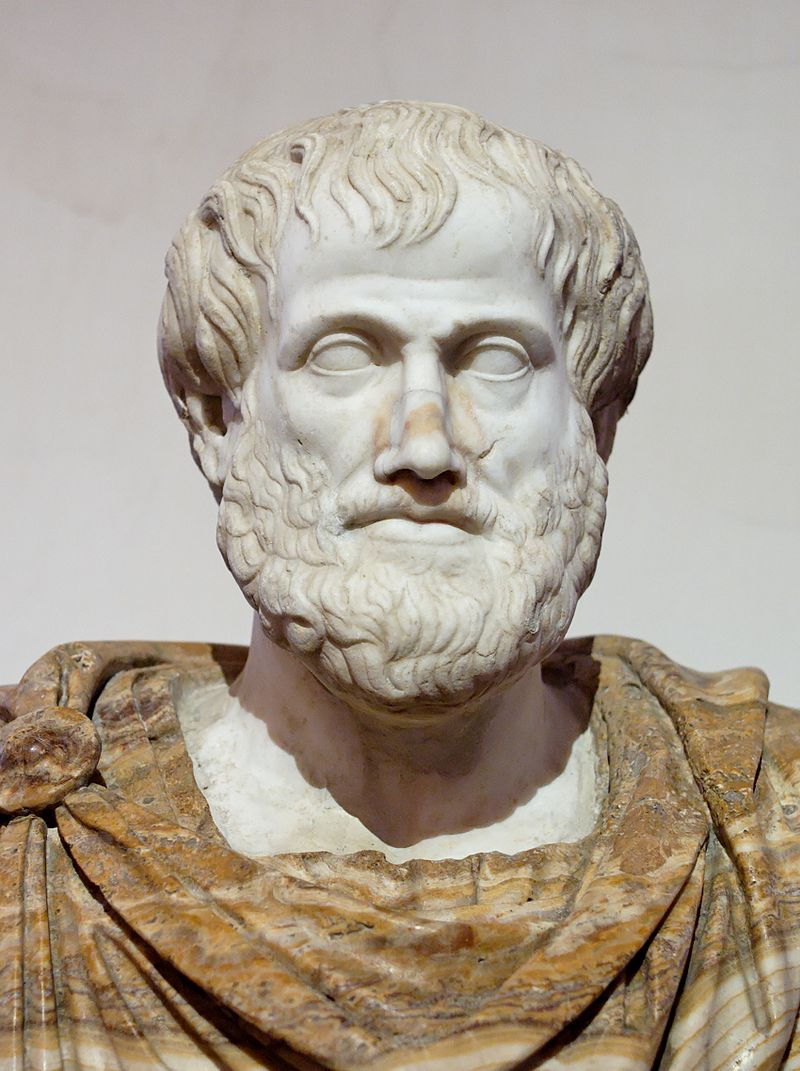Aristotle: It's the nature of heavy things to be at the center of the universe. It's the nature of light things to be up. Done.

## Gravity

Gravity is an attractive force between masses.Its magnitude can be calculated by the following expression:

$$\bbox[15px,border:4px solid red]{F_\textrm{gravity} = G\frac{m_1 m_2}{r^2}}$$

$G$ is a constant and equals $6.674 \times 10^{-11}\;\rm N\cdot m^2/kg^2$

### DirectionsThe force acting on mass 1 will point away from mass 1, towards mass 2. We can express this direction with a unit vector $\mathbf{\hat{r}}$ which points in the direction from mass 1 to mass 2.Three masses are in deep space. The two large masses, each of mass $m$, are located equal distances away from the x axis both at x = 0. A third mass, $m_0$ is located on the x axis as shown. In which direction is the net gravitational force acting on $m_0$?

1. +x
2. –x
3. +y
4. –x
5. A mix of x and y.
6. No direction since the net force is zero.Find an expression (in terms of $d$) that gives the net force on the small mass as a function of position along the x axis. (Let $m_1 = m_2$) Where will it be the largest? And the smallest?

page

## Near the surface of the Earth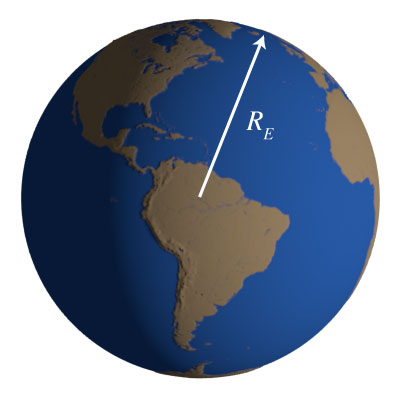The radius of the earth is a big number. When we put it in the denominator of the force function, and square it we get really big number. $${F_\textrm{G} = G\frac{M_E m}{R_E^2}} = G \frac{M_E m}{6378000^2} = G \frac{M_E m}{40678884000000}$$ And so, when we talk about going 1 km up into the sky, we're really not changing the denominator of this equation all that much.

Compare: $$\frac{1}{6378000^2}\; \;\textrm{and} \;\; \frac{1}{6379000^2}$$ To: $$\frac{40678884000000}{40691641000000} = 0.999686$$

### little g

$$F_\textrm{G} = G\frac{M_E m}{R_E^2} = \underbrace{\left( \frac{G M_E}{R_E^2}\right)}_g m = mg$$

### Does little g change?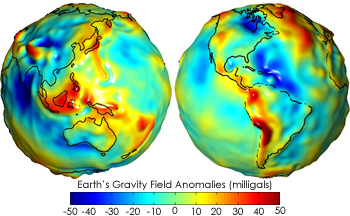The acceleration due to gravity changes by small amounts in different locations around the earth.

Cityvalue of $g$
New York City9.8036
London9.81599
Mexico City9.77628
Copenhagen9.82057

The mass is not uniformly distributed: Some parts of the earth are denser than other parts. This leads to more mass underneath and a higher g value.The earth is not a perfect sphere: The earth is a little wider than it is tall. Thus, if you're on the equator, you're a little bit further away from the center than if you're at the pole.

The earth is rotating: centripetal accelerations exist for objects on rotating surfaces.

How does the rotation effect the value of $g$?

1. The rotation of the earth increases $g$
2. The rotation of the earth decreases $g$
3. The rotation of the earth doesn't affect little g

### Satellites in Orbit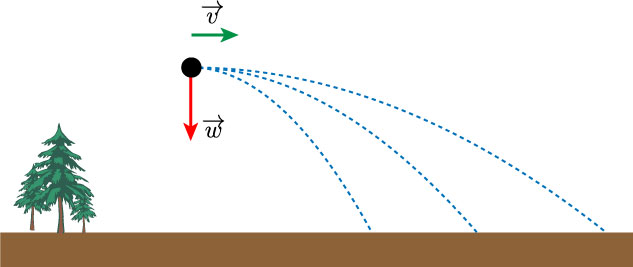Thus, putting an object into orbit just requires a fast enough initial velocity in the right direction.### What about the force vectors?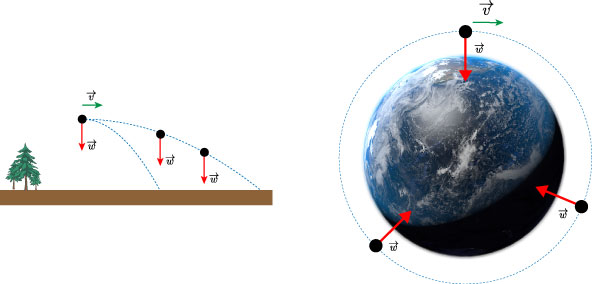Near the surface of the earth we assumed the vectors were always point 'down'. However when we look at the earth as sphere, we see that the force vectors for the weight of an object actually point towards the center of the earth.

## Potential Energy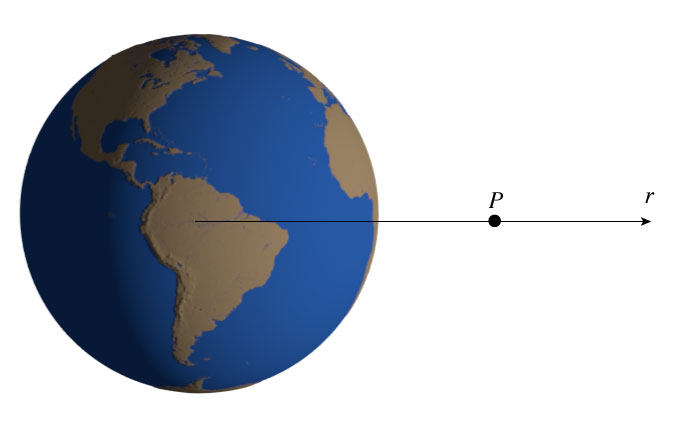Find the work done on an object by gravity as it moves from point $P$ to infinity, where the potential energy is defined as 0. $$W = \int_R^\infty \mathbf{F(r)} \cdot d\mathbf{r}$$ The force is always against the displacement, so $$\mathbf{F(r)} \cdot d\mathbf{r} = -\frac{G M_E m}{r^2} dr$$ We can pull the constants out of the integral, and we are left with something very simple to integrate: $$W = - G M_E m \int_R^\infty \frac{1}{r^2}dr = \left[ \frac{G M_E m}{r} \right]^\infty_R$$Evaluating at the limits of $\infty$ and $R$, we see that the work done is: $$W = \left[ \frac{G M_E m}{r} \right]^\infty_R = 0 - \frac{G M_E m }{R} = - \frac{G M_E m }{R}$$ From the original definition of work and potential energy: $$\Delta U = U_f - U_i = -W$$ thus, since $U_\infty = 0$ we can say: $U = W$ And now we have a potential energy function for masses at a distance $r$ from the center of the Earth: $$\bbox[15px,border:4px solid red]{U = -\frac{GM_e m}{r}}$$

### Potential Plot### Escape Speed

High high do you have to throw something before it never comes back down? or how fast do you need to throw something to get this high?

Essentially we need to give it enough kinetic energy to just reach infinity and then stop. Thus at infinity is has no potential and no kinetic, so total mechanical energy is zero. That means at the surface, the total mechanical energy was also zero: $$K + U = \frac{1}{2}mv^2 + \left(-\frac{G M_E m}{R_E^2} \right) = 0$$ Solve this for $v$: $$v = \sqrt{\frac{2 G M_E}{R_E}}$$

#### Example speeds:

Celestial Body Escape Speed (km/s)
Earth 11.2
Moon 2.38
Sun 618
Moon 2.38
Phobos 11.4 m/s

## Orbits

In the case of an orbiting body, i.e. a planet around the sun, or a moon around a planet, or a satellite around the earth, the only force acting is the centrally directed gravitational attraction between the two bodies.

$$\sum F = F_\textrm{grav} = G \frac{m_1 m_2}{r^2}$$

Using the second law, we can write:

$$F = G \frac{m_1 m_2}{r^2} = m_2a = m_2 \frac{v^2}{r}$$

Now we can predict some universal behaviors of orbiting objects.

For example, for an object orbiting the earth, we can write:

$$G \frac{M_E m}{r^2} = m \frac{v^2}{r}$$

We can solve this for $v$, the speed of the orbiting object:

$$v= \sqrt{\frac{G M_E}{r}}$$

This gives the speed of an object for a given orbital distance.

!!!Notice that there is no $m$ in this equation!!!

Now we can use the gravitational force to calculate the expected period:

$$v = \sqrt{\frac{G M_e}{r}} = \frac{2 \pi r}{T}$$

which can be solved for $T$:

$$T = \frac{2\pi r^{3/2}}{\sqrt{G M_E}}$$Kepler figured out a version of this relationship before Newton: $$T \propto r^{3/2}$$ The period is proportional to the three-halfs power of the orbital radius.

How fast will an object need to travel to orbit the earth at its surface?### Kepler's Laws of Planetary Motion

1. The orbit of a planet is an ellipse with the Sun at one of the two foci.

2. A line segment joining a planet and the Sun sweeps out equal areas during equal intervals of time

3. The square of the period is proportional to the cube of the radius

### Kepler's Harmony of the World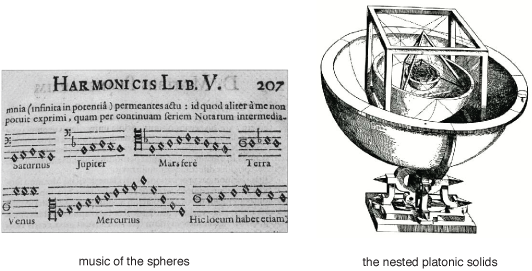### A very special orbit

There will be an orbital distance where the period of the orbiting satellite exactly matches the rotation of the earth.

List of geosynchronous satellites

Find the position/radius for a geosynchronous orbit around the earth.